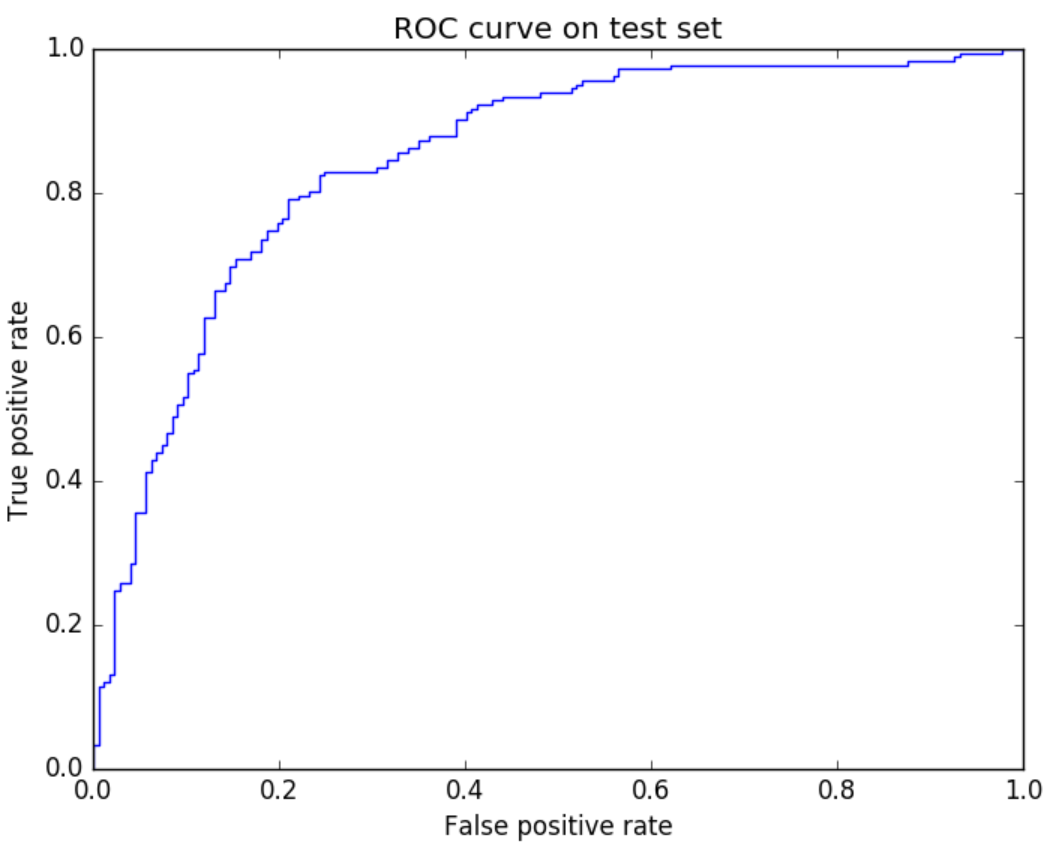Version: 0.11.0

# Twitter Sentiment Classification using Vowpal Wabbit in SynapseML

In this example, we show how to build a sentiment classification model using Vowpal Wabbit (VW) in SynapseML. The data set we use to train and evaluate the model is Sentiment140 twitter data. First, we import a few packages that we need.

import osimport reimport urllib.requestimport numpy as npimport pandas as pdfrom zipfile import ZipFilefrom bs4 import BeautifulSoupfrom pyspark.sql.functions import udf, rand, when, colfrom pyspark.sql.types import StructType, StructField, DoubleType, StringTypefrom pyspark.ml import Pipelinefrom pyspark.ml.feature import CountVectorizer, RegexTokenizerfrom synapse.ml.vw import VowpalWabbitClassifierfrom synapse.ml.train import ComputeModelStatisticsfrom pyspark.mllib.evaluation import BinaryClassificationMetricsimport matplotlib.pyplot as plt
from pyspark.sql import SparkSession# Bootstrap Spark Sessionspark = SparkSession.builder.getOrCreate()
# URL to download the sentiment140 dataset and data file namesDATA_URL = "https://mmlspark.blob.core.windows.net/publicwasb/twittersentimenttrainingandtestdata.zip"TRAIN_FILENAME = "training.1600000.processed.noemoticon.csv"TEST_FILENAME = "testdata.manual.2009.06.14.csv"# Folder for storing the downloaded dataDATA_FOLDER = "data"# Data column namesCOL_NAMES = ["label", "id", "date", "query_string", "user", "text"]# Text encoding type of the dataENCODING = "iso-8859-1"

## Data Preparation​

We use Sentiment140 twitter data which originated from a Stanford research project to train and evaluate VW classification model on Spark. The same dataset has been used in a previous Azure Machine Learning sample on twitter sentiment prediction. Before using the data to build the classification model, we first download and clean up the data.

def download_data(url, data_folder=DATA_FOLDER, filename="downloaded_data.zip"):    """Download and extract data from url"""    data_dir = "./" + DATA_FOLDER    if not os.path.exists(data_dir):        os.makedirs(data_dir)    downloaded_filepath = os.path.join(data_dir, filename)    print("Downloading data...")    urllib.request.urlretrieve(url, downloaded_filepath)    print("Extracting data...")    zipfile = ZipFile(downloaded_filepath)    zipfile.extractall(data_dir)    zipfile.close()    print("Finished data downloading and extraction.")download_data(DATA_URL)

Let's read the training data into a Spark DataFrame.

df_train = pd.read_csv(    os.path.join(".", DATA_FOLDER, TRAIN_FILENAME),    header=None,    names=COL_NAMES,    encoding=ENCODING,)df_train = spark.createDataFrame(df_train, verifySchema=False)

We can take a look at the training data and check how many samples it has. We should see that there are 1.6 million samples in the training data. There are 6 fields in the training data:

• label: the sentiment of the tweet (0.0 = negative, 2.0 = neutral, 4.0 = positive)
• id: the id of the tweet
• date: the date of the tweet
• query_string: The query used to extract the data. If there is no query, then this value is NO_QUERY.
• user: the user that tweeted
• text: the text of the tweet
df_train.limit(10).toPandas()
print("Number of training samples: ", df_train.count())

Before training the model, we randomly permute the data to mix negative and positive samples. This is helpful for properly training online learning algorithms like VW. To speed up model training, we use a subset of the data to train the model. If training with the full training set, typically you will see better performance of the model on the test set.

df_train = (    df_train.orderBy(rand())    .limit(100000)    .withColumn("label", when(col("label") > 0, 1.0).otherwise(0.0))    .select(["label", "text"]))

## VW SynapseML Training​

Now we are ready to define a pipeline which consists of feature engineering steps and the VW model.

# Specify featurizerstokenizer = RegexTokenizer(inputCol="text", outputCol="words")count_vectorizer = CountVectorizer(inputCol="words", outputCol="features")# Define VW classification modelargs = "--loss_function=logistic --quiet --holdout_off"vw_model = VowpalWabbitClassifier(    featuresCol="features", labelCol="label", passThroughArgs=args, numPasses=10)# Create a pipelinevw_pipeline = Pipeline(stages=[tokenizer, count_vectorizer, vw_model])

With the prepared training data, we can fit the model pipeline as follows.

vw_trained = vw_pipeline.fit(df_train)

## Model Performance Evaluation​

After training the model, we evaluate the performance of the model using the test set which is manually labeled.

df_test = pd.read_csv(    os.path.join(".", DATA_FOLDER, TEST_FILENAME),    header=None,    names=COL_NAMES,    encoding=ENCODING,)df_test = spark.createDataFrame(df_test, verifySchema=False)

We only use positive and negative tweets in the test set to evaluate the model, since our model is a binary classification model trained with only positive and negative tweets.

print("Number of test samples before filtering: ", df_test.count())df_test = (    df_test.filter(col("label") != 2.0)    .withColumn("label", when(col("label") > 0, 1.0).otherwise(0.0))    .select(["label", "text"]))print("Number of test samples after filtering: ", df_test.count())
# Make predictionspredictions = vw_trained.transform(df_test)predictions.limit(10).toPandas()
# Compute model performance metricsmetrics = ComputeModelStatistics(    evaluationMetric="classification", labelCol="label", scoredLabelsCol="prediction").transform(predictions)metrics.toPandas()
# Utility class for plotting ROC curve (https://stackoverflow.com/questions/52847408/pyspark-extract-roc-curve)class CurveMetrics(BinaryClassificationMetrics):    def __init__(self, *args):        super(CurveMetrics, self).__init__(*args)    def get_curve(self, method):        rdd = getattr(self._java_model, method)().toJavaRDD()        points = []        for row in rdd.collect():            points += [(float(row._1()), float(row._2()))]        return pointspreds = predictions.select("label", "probability").rdd.map(    lambda row: (float(row["probability"]), float(row["label"])))roc_points = CurveMetrics(preds).get_curve("roc")# Plot ROC curvefig = plt.figure()x_val = [x for x in roc_points]y_val = [x for x in roc_points]plt.title("ROC curve on test set")plt.xlabel("False positive rate")plt.ylabel("True positive rate")plt.plot(x_val, y_val)# Use display() if you're on Azure Databricks or you can do plt.show()plt.show()

You should see an ROC curve like the following after the above cell is executed.